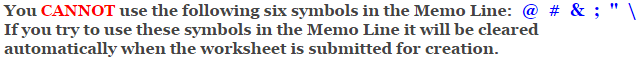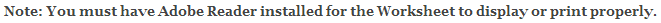# Algebra 1 - Linear Equations Worksheets

## Finding Slope and Y-intercept from an Equation Worksheets

This Linear Equations Worksheet will produce problems for practicing finding the slope and Y-intercept from an equation.

### Language for the Linear Equations Worksheet

 English German Albanian Spanish Swedish Italian French Turkish Polish Norwegian

### Memo Line for the Linear Equations Worksheet

You may enter a message or special instruction that will appear on the bottom left corner of the Linear Equations Worksheet.### Linear Equations Worksheet Answer Page

Now you are ready to create your Linear Equations Worksheet by pressing the Create Button.

 Recommended Videos

If You Experience Display Problems with Your Math Worksheet